Updating search results...

# 25 Results

View
Selected filters:
• NC.Math.8.G.6Conditional Remix & Share Permitted
CC BY-NC-SA
Rating
0.0 stars

This 8th grade Math&nbsp;parent guide&nbsp;explains the content in straightforward terms so they can support their children&rsquo;s learning at home and will&nbsp;encourage&nbsp;caretaker engagement&nbsp;with lessons.

Subject:
Mathematics
Material Type:
Reference Material
Author:
Kelly Rawlston
Letoria Lewis
01/10/2022Conditional Remix & Share Permitted
CC BY-NC-SA
Rating
0.0 stars

Our Teacher Guides are meant to support the use of our online course and unit content. Please use these to accompany the use of our content and for ideas to support struggling learners, those needing extension and for additional resources.

Subject:
Mathematics
Material Type:
Curriculum
Teaching/Learning Strategy
Author:
Kelly Rawlston
Letoria Lewis
05/16/2022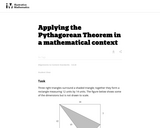Unrestricted Use
CC BY
Rating
0.0 stars

This task requires students to apply the Pythagorean Theorem.

Subject:
Mathematics
Material Type:
Activity/Lab
Provider:
Illustrative Mathematics
Provider Set:
Illustrative Mathematics
Author:
Illustrative Mathematics
05/01/2012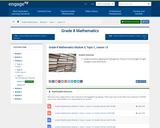Conditional Remix & Share Permitted
CC BY-NC-SA
Rating
0.0 stars

In this lesson, students practice applying the Pythagorean Theorem to find lengths of right triangles in two dimensions.

Subject:
Mathematics
Material Type:
Lesson Plan
Provider:
EngageNY
02/16/2017Rating
0.0 stars

This document provides sample performance tasks/assessment items for Common Core State Standards Grade 8 Math provided by the Louisiana Department of Eduation. Both questions and exemplary responses are included.

Subject:
Mathematics
Material Type:
Activity/Lab
Assessment
Provider:
Louisiana Department of Education
Author:
Louisiana Department of Education
02/26/2019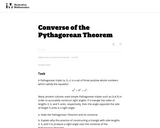Unrestricted Use
CC BY
Rating
0.0 stars

This task is for instruction purposes. Part (b) is subtle and the solution presented here uses a "dynamic" view of triangles with two side lengths fixed. This helps pave the way toward what students will see later in trigonometry but some guidance will likely be needed in order to get students started on this path.

Subject:
Mathematics
Material Type:
Activity/Lab
Provider:
Illustrative Mathematics
Provider Set:
Illustrative Mathematics
Author:
Illustrative Mathematics
08/17/2012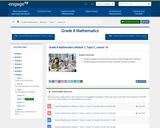Conditional Remix & Share Permitted
CC BY-NC-SA
Rating
0.0 stars

For this lesson, students explain a proof of the converse of the Pythagorean Theorem. Students apply the theorem and its converse to solve problems.

Subject:
Mathematics
Material Type:
Lesson Plan
Provider:
EngageNY
02/24/2017Conditional Remix & Share Permitted
CC BY-NC
Rating
0.0 stars

Introduction to the Pythagorean Theorem through reading a story about the boy Pythagoras.&nbsp;This lesson was developed by Michael Tinker as part of their completion of the North Carolina Global Educator Digital Badge program. This lesson plan has been vetted at the local and state level for standards alignment, Global Education focus, and content accuracy.

Subject:
Mathematics
Material Type:
Lesson Plan
Author:
Melody Casey
02/19/2020Conditional Remix & Share Permitted
CC BY-NC
Rating
0.0 stars

Discussion about how the Pythagorean Theorem works and what it does for us.&nbsp;This lesson was developed by Michael Tinker as part of their completion of the North Carolina Global Educator Digital Badge program. This lesson plan has been vetted at the local and state level for standards alignment, Global Education focus, and content accuracy.

Subject:
Mathematics
Material Type:
Lesson Plan
Author:
Melody Casey
02/19/2020Rating
0.0 stars

For this task, students use Pythagorean Triples to solve problems.

Subject:
Mathematics
Material Type:
Activity/Lab
Provider:
NRICH
03/23/2017Rating
0.0 stars

Students will learn about translations, reflections, and rotations in the plane and, more importantly, how to use them to precisely define the concept of congruence.

Subject:
Mathematics
Material Type:
Unit of Study
Provider:
EngageNY
Author:
EngageNY
02/26/2019Rating
0.0 stars

Students will learn about dilation and similarity and apply that knowledge to a proof of the Pythagorean Theorem based on the Angle-Angle criterion for similar triangles.

Subject:
Mathematics
Material Type:
Unit of Study
Provider:
EngageNY
Author:
EngageNY
02/26/2019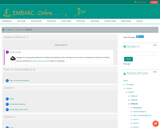Conditional Remix & Share Permitted
CC BY-NC-SA
Rating
0.0 stars

Grade 8 Module 3: Similarity. Contains 14 Lessons.

Subject:
Mathematics
Material Type:
Module
Provider:
EMBARC.Online
08/13/2019Rating
0.0 stars

This lesson will explores why is c2 = a2 + b2. The students will watch a dynamic, geometric "proof without words" of the Pythagorean Theorem, and then will be asked to explain the proof.

Subject:
Mathematics
Material Type:
Activity/Lab
Provider:
NCTM Illuminations Resources for Teaching Math
02/26/2019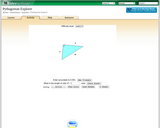Rating
0.0 stars

For this interactive, students study the nature of the Pythagorean Theorem by calculating the measure of the unknown side.

Subject:
Mathematics
Material Type:
Interactive
Provider:
Shodor Education Foundation
03/23/2017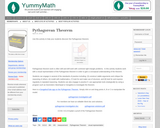Rating
0.0 stars

For this activity, students work with a visual representation of the Pythagorean theorem in order to gain a conceptual understanding of the theorem.

Subject:
Mathematics
Material Type:
Activity/Lab
Provider:
YummyMath
04/27/2017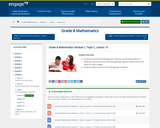Conditional Remix & Share Permitted
CC BY-NC-SA
Rating
0.0 stars

For this lesson, students know that the Pythagorean Theorem can be interpreted as a statement about the areas of similar geometric figures constructed on the sides of a right triangle. Students explain a proof of the Pythagorean Theorem.

Subject:
Mathematics
Material Type:
Lesson Plan
Provider:
EngageNY
02/24/2017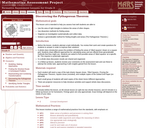Only Sharing Permitted
CC BY-NC-ND
Rating
0.0 stars

This lesson unit is intended to help teachers assess how well students are able to: use the area of right triangles to deduce the areas of other shapes; use dissection methods for finding areas; organize an investigation systematically and collect data; deduce a generalizable method for finding lengths and areas (The Pythagorean Theorem.)

Subject:
Geometry
Mathematics
Material Type:
Assessment
Lesson Plan
Provider:
Shell Center for Mathematical Education
Provider Set:
Mathematics Assessment Project (MAP)
06/24/2019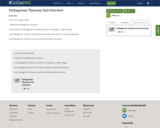Unrestricted Use
Public Domain
Rating
0.0 stars

In this unit students will:-Understand Pythagorean Theorem-Use Converse of&nbsp;Pythagorean Theorem to prove if a triangle is a right triange-Use Pythagorean Theorem to find distance between two points on a coordinate plane-Use Pythagorean Theorem to solve real world problem situations

Subject:
Mathematics
Material Type:
Unit of Study
Author:
Lindsey Fodrie
06/21/2021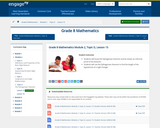Conditional Remix & Share Permitted
CC BY-NC-SA
Rating
0.0 stars

In this lesson, students will know the Pythagorean theorem and be shown an informal proof of the theorem. Students will use the Pythagorean theorem to find the length of the hypotenuse of a right triangle.

Subject:
Mathematics
Material Type:
Lesson Plan
Provider:
EngageNY